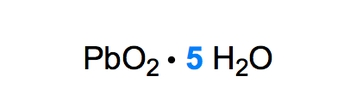## GOB Chemistry

Learn the toughest concepts covered in your GOB - General, Organic, and Biological Chemistry class with step-by-step video tutorials and practice problems.

Ionic Compounds

# Naming Ionic Hydrates

An Ionic Hydrate is an ionic compound that is linked to at least one molecule of H2O.

Naming Ionic Hydrates
1
concept

## Naming Ionic Hydrates2m
Play a video:
in these series of videos will learn what ionic hydrates are and the rules needed to name them. Now we're gonna say ionic hydrates are simply ionic compounds linked to at least one molecule of water. Here we have as our example see you. X h 20 X just represents some unknown number of water molecules that are linked to copper and encore ing together here now. But dot here is what's referring to as the linking or bonding of the water molecules to this Ionic compound. Now that we know what no Ionic hydrate is, let's look at the rules to name them. Some of these rules are pretty familiar to us because, ah, big chunk of it has to do with naming ionic compounds, which we've covered in earlier videos. Now, step 0 to 3 are the same rules as naming ionic compounds. If you don't remember those rules, make sure you go back and take a look at our videos on naming ionic compounds. Step four is new for Step four. We're going to say that the water portion will be called hydrate, but we also have to specify how many of these hydrates are connected to my ionic compound, we're going to say to describe the number of water molecules in a hydrate, we now use numerical prefixes. Now these numerical prefixes go from mono to Decca mono, meaning one and then dies to try is three. Tetra is four. Some of these terms were kind of familiar with. Pentagon has five sides, So Penta is five. Hexagon has six sides. Hep tha. We may not have been familiar with that because that's not a term that usually seen, so I have to. It's seven. Octagon eight now Nana Nana is nine and Decca is 10. So now that we know how to identify Ionic hydrates and we've learned the basic rules for naming them, let's take a look at some questions and put to what we learned into practice.2
example

## Naming Ionic Hydrates Example 12m
Play a video:
in this example Question. We're told to name the following Ionic Hydrate. All right, so let's do the easy part. First, we have five water molecules here, so five would mean that we're using the numerical prefix of Penta, and the water would be called hydrates. So this is Penta hydrate. Now, if we take a look at the eye on a compound portion, we have led with oxygen with lead. We have an issue, though Lead can have a charge of either two plus or four Plus, remember, lead is not a transition metal, but it and tin have this issue where they can be two plus or four. Plus, If you don't remember that again, go back and take a look at my periodic table videos that deal with charges Now here. Oxygen oxygen is in Group six. A. Remember a few groups? Six. A. Your charge is two minus or minus two, so let's think about this. Oxygen is minus two. There are two oxygen's within the formula, so collectively, the oxygen's together R minus four for P B 02 to be neutral. This minus four has to be counterbalanced or canceled out by what by a plus four. So the plus four of the lead cancels out the minus four from the two oxygen's, thereby giving us a neutral ionic compound. So that would mean that we're dealing with for our our Ionic compound lead. Four, Remember? Uses Roman numerals because it has multiple charges. Led four Oxygen's based name is Ox, and then we put it at the end. So lead for oxide Penta hydrate. So this one was tricky, but this would be the name of our ionic hydrate.
3
Problem

Give the systematic name from the given formula:K2Cr2O7 ∙ 3 H2O

4
Problem

Write the chemical formula for the following compound:Calcium selenide hexahydrate